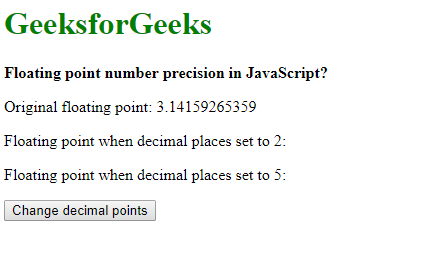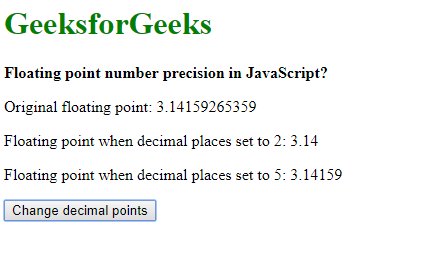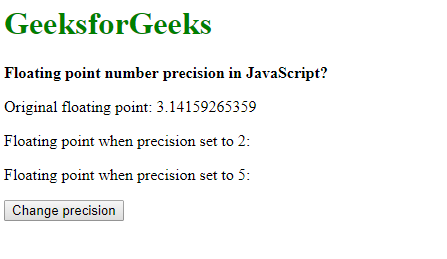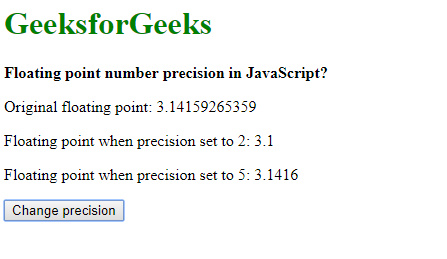# Floating point number precision in JavaScript

The representation of floating points in JavaScript follows the IEEE-754 format. It is a double precision format where 64 bits are allocated for every floating point. The displaying of these floating values could be handled using 2 methods:

Using toFixed() Method: The number of decimal places in float values can be set using the toFixed() method. This method converts the number into a string, keeping the specified number of digits after the point. If no value is passed as a parameter, then it takes 0 as default value i.e. no decimal points are displayed.

Syntax:

`number.toFixed(digits)`

Example:

 ` ` `<``html``> ` `     `  `<``head``> ` `    ``<``title``> ` `        ``Floating point number precision ` `        ``in JavaScript? ` `    `` ` ` ` ` `  `<``body``> ` `    ``<``h1` `style``=``"color: green"``> ` `        ``GeeksforGeeks ` `    `` ` `     `  `    ``<``b``> ` `        ``Floating point number precision ` `        ``in JavaScript? ` `    `` ` `     `  `    ``<``p``> ` `        ``Original floating point: 3.14159265359 ` `    `` ` `     `  `    ``<``p``> ` `        ``Floating point when decimal places set ` `        ``to 2: <``span` `class``=``"output-2"``> ` `    `` ` `     `  `    ``<``p``> ` `        ``Floating point when decimal places set ` `        ``to 5: <``span` `class``=``"output-5"``> ` `    `` ` ` `  `    ``<``button` `onclick``=``"setDecimalPlaces()"``> ` `        ``Change decimal points ` `    `` ` `     `  `    ``<``script` `type``=``"text/javascript"``> ` `        ``function setDecimalPlaces() { ` `            ``pi = 3.14159265359; ` `            ``twoPlaces = pi.toFixed(2); ` `            ``fivePlaces = pi.toFixed(5); ` ` `  `            ``document.querySelector('.output-2').textContent ` `                    ``= twoPlaces; ` `            ``document.querySelector('.output-5').textContent ` `                    ``= fivePlaces; ` `        ``} ` `    `` ` ` ` ` `  `                     `

Output:

• Before clicking the button:• After clicking the button:Using toPrecision() Method: The number of total digits in float values can be set using the toPrecision() method. This method converts the number into a string, keeping the total number of digits of the value as specified and rounding them to the nearest number. If no value is passed as a parameter, then the function acts as a toString() function effectively returning the value passed as a string.

Syntax:

`number.toPrecision(precision)`

Example:

 ` ` `<``html``> ` `     `  `<``head``> ` `    ``<``title``> ` `        ``Floating point number precision ` `        ``in JavaScript? ` `    `` ` ` ` ` `  `<``body``> ` `    ``<``h1` `style``=``"color: green"``> ` `        ``GeeksforGeeks ` `    `` ` `     `  `    ``<``b``> ` `        ``Floating point number precision ` `        ``in JavaScript? ` `    `` ` `     `  `    ``<``p``> ` `        ``Original floating point: 3.14159265359 ` `    `` ` `     `  `    ``<``p``> ` `        ``Floating point when precision set to ` `        ``2: <``span` `class``=``"output-2"``> ` `    `` ` `     `  `    ``<``p``> ` `        ``Floating point when precision set to ` `        ``5: <``span` `class``=``"output-5"``> ` `    `` ` ` `  `    ``<``button` `onclick``=``"setPrecision()"``> ` `        ``Click to check ` `    `` ` `     `  `    ``<``script` `type``=``"text/javascript"``> ` `        ``function setPrecision() { ` `            ``pi = 3.14159265359; ` `            ``twoPlaces = pi.toPrecision(2); ` `            ``fivePlaces = pi.toPrecision(5); ` ` `  `            ``document.querySelector('.output-2').textContent ` `                    ``= twoPlaces; ` `            ``document.querySelector('.output-5').textContent ` `                    ``= fivePlaces; ` `        ``} ` `    `` ` ` ` ` `  `                     `

Output:

• Before clicking the button:• After clicking the button:My Personal Notes arrow_drop_upIm listening

If you like GeeksforGeeks and would like to contribute, you can also write an article using contribute.geeksforgeeks.org or mail your article to contribute@geeksforgeeks.org. See your article appearing on the GeeksforGeeks main page and help other Geeks.

Please Improve this article if you find anything incorrect by clicking on the "Improve Article" button below.